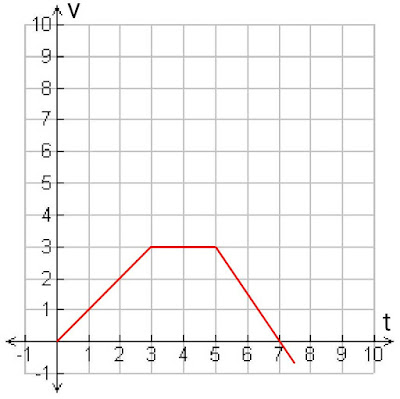In physics, Acceleration means we simply understand "speed up", We all have seen in motor vehicles there is an accelerator which is used for speeding up the vehicle. We can also say that we use accelerator to accelerate the vehicle.

Before we start discussion about acceleration first we should know in short, What is Velocity?

Velocity is vector quantity which indicates time, direction and displacement of a body. The SI unit of velocity is m/s (meter per second).

## Acceleration Definition

The rate of change of velocity of a body with the time is known as acceleration.

Let's try to understand easy way what is acceleration? While riding in a car, bus or train we all have experienced that when breaks are applied we get pushed ahead, when it takes sharp right turn we get  pushed towards left and when it speed up we pushed backward.

These are all situations of acceleration. In simple words, there will be acceleration whenever the velocity changes.

#### Examples of Acceleration

These are some perfect examples acceleration discussed below,

• When an aeroplane takes off from an airport the speed of aeroplane increases gradually. And this change in speed called acceleration.
• When a train slows down its speed to stop at the station. There is acceleration as the speed of train decreasing.
• When a football player kicks the football towards the goal. The football starts moving towards the goal faster.  This is also an example of acceleration.

## How to Measure Acceleration?

The SI unit of acceleration is meter per second square

SI Unit.           m/s2

Acceleration is a vector as it has both magnitude and direction.

## Acceleration Formula

Acceleration formula can be written as change in velocity upon time. Let's see how the formula comes,

Acceleration = Change is velocity / time

Acceleration = final velocity - initial velocity / time

a = vf - vi / t
a = ∆v / t

Where,
• a is acceleration
• t is the time interval
• Vf is the final velocity
• Vi is the initial velocity
• ∆V is the change in velocity

### Average Acceleration

The average acceleration can be defined as the total change in  velocity is divided into many smaller intervals of time. Average acceleration formula can be written as,

Average acceleration = final velocity - initial velocity / final time - initial time

Average acceleration = Change in velocity / change in time

-a = v2 - v1 / t2 - t1
-a = ∆v / ∆t

Where,
• -a is average acceleration
• V2 is final velocity
• V1 is initial velocity
• t2 is final time taken
• t1 is initial time taken
• ∆v is change in velocity
• ∆t is change in time interval.

Sometimes the change of velocity may remain constant at that time the acceleration also remains constant and this is called Uniform acceleration.

### Instantaneous Acceleration

Instantaneous acceleration is the acceleration of an object at a specific moment of time. The formula for instantaneous acceleration can be written as,

Instantaneous Acceleration = $dv/dt$

## Positive, Negative and  Zero Acceleration

Acceleration can be positive, negative and zero but there are mainly two types of acceleration,

1. Positive Acceleration
2. Deceleration or Negative Acceleration
3. Zero Acceleration

Now, we are going to discuss below the situations when acceleration of a body can be positive, negative or zero. You can understand all the three cases with the help of the velocity-time graph.Velocity Time Graph

Positive Acceleration: As you observed on the above velocity-time graph the velocity of an object is increasing between the time interval of 0-3. In this case the acceleration is positive as the object or body is experiencing positive acceleration.

Zero Acceleration: A body experiences zero acceleration when the velocity does not change and remains constant. On the above graph you can see between the time interval of 3-5 there is no change in velocity and it is constant. So, the slope of the velocity curve during this time interval is 0.

Negative Acceleration: Negative acceleration is also known as Deceleration or Retardation. When the velocity of an object decrease with respect to time the body experiences negative acceleration. The direction of the negative acceleration is always opposite to the direction in which the body is moving.

In the above graph the time interval between 5-7 is decreasing, hence the body is experiencing negative acceleration and the slope on the velocity time graph is negative.

## Acceleration in Relation to Force

According to Newton's second law of motion the force on an object equals the mass times acceleration which can be written as the equation below,

Force = mass × acceleration

F = ma

If the force and mass of an object is given we can easily find out the acceleration with the help of the equation above. The formula is,

Acceleration = force / mass

a = F/m